Function Repository Resource:

# AlgebraicReplace

Replace all occurrences of an algebraic subexpression with a new symbol

Contributed by: Daniel Lichtblau (Wolfram Research)
 ResourceFunction["AlgebraicReplace"][expr,reps,repvars] rewrites expr, replacing each occurrence of an element of reps with the corresponding element of repvars. ResourceFunction["AlgebraicReplace"][expr,reps,repvars,vars] rewrites expr, burrowing inside any subexpression that is not a polynomial in vars.

## Details

As the name implies, ResourceFunction["AlgebraicReplace"] provides an algebraic form of polynomial replacement as opposed to requiring matching of patterns as used by ReplaceAll and related functions. For example, ordinary replacement of x y by z in the monomial x2y3 will have no effect, whereas AlgebraicReplace will return y z2.
ResourceFunction["AlgebraicReplace"] uses PolynomialQ to determine whether to burrow into subexpressions. If vars is not specified, it will use Variables to determine them.

## Examples

### Basic Examples (1)

Algebraically replace xy by a new variable z in a bivariate polynomial:

 In:=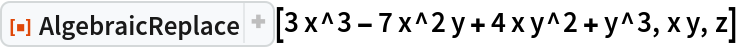Out=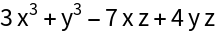### Scope (2)

AlgebraicReplace works with non-polynomial expressions:

 In:=Out=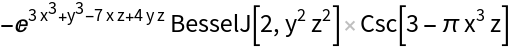Use several replacements at once:

 In:=Out=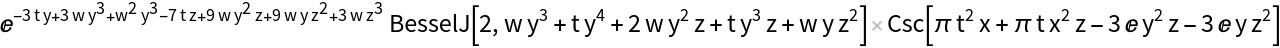### Properties and Relations (2)

ReplaceAll only replaces literal matches:

 In:=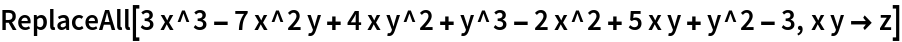Out=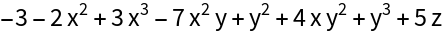AlgebraicReplace rewrites all monomials containing powers of xy to have powers of z:

 In:=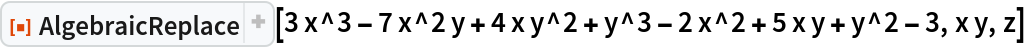Out=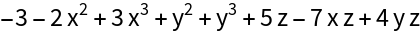AlgebraicReplace with a set of reducing polynomials polys and replacement variables vars has behavior similar to PolynomialReduce with polys-vars:

 In:=Out=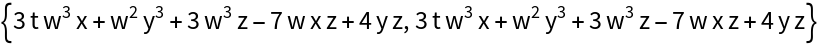This is not canonical insofar as the reduction is not done with a Gröbner basis, so results can depend on ordering of inputs:

 In:=Out=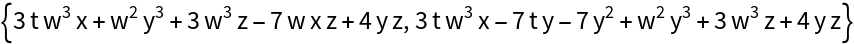If we compute a Gröbner basis then the result is canonical for the given variable ordering:

 In:=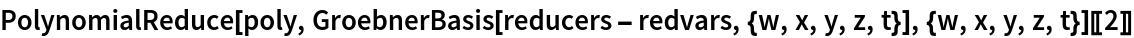Out=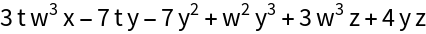Reversing the reduction polynomials does not change the result of PolynomialReduce when a Gröbner basis is used:

 In:=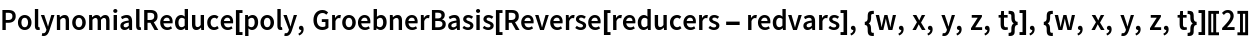Out=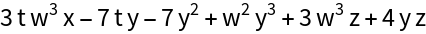### Possible Issues (2)

If the underlying variables are omitted, AlgebraicReplace might not recognize what are the correct ones:

 In:=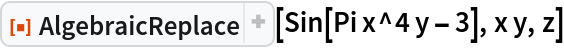Out=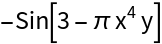Specify x and y as variables to get the desired replacement:

 In:=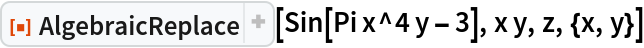Out=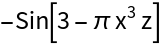As seen in the "Properties and Relations" examples, AlgebraicReplace does not compute and use a Gröbner basis. Changing the order of the reducer inputs can affect the result.

## Version History

• 1.0.1 – 26 May 2023
• 1.0.0 – 08 May 2023

## Author Notes

This functionality is often requested on MSE, Wolfram Community and older forums as well. It could benefit from a better get-all-variables function. Another task for another day…